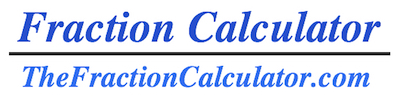Welcome to the Fraction Calculator. Please choose your fraction problem below.

Fraction {} Fraction
 + - × ÷

Integer {} Integer
 + - × ÷

Integer {} Fraction
 + - × ÷

Fraction {} Integer
 + - × ÷

Mixed {} Fraction
 + - × ÷

Fraction {} Mixed
 + - × ÷

Mixed {} Mixed
 + - × ÷

Mixed {} Integer
 + - × ÷

Integer {} Mixed
 + - × ÷

Thank you for using the Fraction Calculator to find and calculate fraction problems.

Fraction Directory
For detailed instructions, step-by-step explanations, and solutions to all kinds of fraction problems, please see our directory.

Here are some examples of fraction problems that we have explained and answered:

Pie Chart of 2/3

What is half of 3/4?

What is the denominator of 7 as a repeating decimal?

What is a quarter of 1/4?

1/2 times what equals 1/2?

What divided by 1/4 equals 3/5?

What is 2 over 3 as a percentage?

How to calculate 3/4 plus 1/9

Is 1/2 greater than 1/3?

What is 4.56 as a fraction?

How to calculate 3/7 divided by 4/5

Equivalent Fractions of 3/9

What is the factorial of 6?

1/2 divided by what equals 3/4?

Reduce 5/25

What times 1/3 equals 1/2?

1/3 minus what equals 1/5?

4/9 Rounded to the Nearest Hundredth

What is 1/4 of 1/2?

3/4 Rounded to the Nearest Tenth

What is 4/5 as a decimal?

50/24 Rounded to the Nearest Whole Number

3/4 plus what equals 4/5?

Greatest Common Factor (GCF) of 2, 4 and 6

What is 3/2 as a Mixed Number?

Least Common Denominator (LCD) of 3, 9, and 20

What is 1 3/4 as a Decimal?

What plus 3/4 equals 2/5?

5 5/6 as a Percent

2 3/4 Rounded to the Nearest Half

What is 9 3/4 as an Improper Fraction?

3 3/4 Rounded to the Nearest Whole Number

How to calculate 5/6 times 3/2

What is the Quotient of 6/9?

What is the remainder of 4/5?

What is 48/52 simplified?

How to calculate 4/5 minus 3/4

How to Write 5/9 in Words

What minus 1/4 equals 1/3?